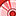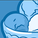# App Development

Announcements
April 22, 2PM EST: Learn about GeoOperations in Qlik Sense SaaS READ MORE
cancel
Showing results for
Did you mean:Contributor

FOR(J=with some condition)

TAB1:

A,B,C,D,E

SQL

SELECT A,B,C, D,SUM(X) as E from v_DB.DB_TAB1 Group by A,B,C,D;

TAB2:

A,B,C,D,X,Y,Z

SELECT * from v_DB.DB_TAB2;

LEFT JOIN(TAB1)

A,B,C,D,

Sum(X),

SUM(if(Y=1,X,0)) as SUM_TYPE1,

SUM((if(Y=2,X,0)) as SUM_TYPE2,

RESIDENT TAB2;

Group by A, B,C,D

Next J;

DROP table TAB2;

1 Solution

Accepted SolutionsContributor
Author

Hello ,

it works when i have put the left join jus after the loop ,

LEFT JOIN(TAB1)

A,B,C,D,

Sum(X),

SUM(if(Y=1,X,0)) as SUM_TYPE1,

SUM((if(Y=2,X,0)) as SUM_TYPE2,

RESIDENT TAB2;

Group by A, B,C,D

3 RepliesSpecialist

Is there any chance that you are loading a table in your load script with the fields A,B,C,D,X,Y,Z prior to this section of code running?  If Qlik encounters a load for a table with the same columns as a previously loaded table, it will concatenate the subsequent table to the first table.  You can prevent this with a Noconcatenate statement before loading TAB2.

Try this:

Noconcatenate

TAB2:

A,B,C,D,X,Y,Z

SELECT * from v_DB.DB_TAB2;MVP & Luminary

Joins within a loop are very problematic and will mostly don't work - because already in the second iteration the join will be applied on the extra added fields from the first iteration. Further the new added fields change the table-structure and therefore the second iteration won't load the data within the origin defined table else Qlik creates a new one by appending a continuous number to the table-name.

I don't want to say that's impossible to use such an approach - you may apply a forced concatenation and/or renaming fields and tables for and back and/or using some intermediated steps with extra stored/loaded tables and similar measures but I wouldn't recommend this direction because nearly always are better and easier approaches possible - especially from a performance point of view are such outside-loops very slow if it comes to many iterations.

- MarcusContributor
Author

Hello ,

it works when i have put the left join jus after the loop ,

LEFT JOIN(TAB1)

A,B,C,D,

Sum(X),

SUM(if(Y=1,X,0)) as SUM_TYPE1,

SUM((if(Y=2,X,0)) as SUM_TYPE2,

RESIDENT TAB2;

Group by A, B,C,DTags